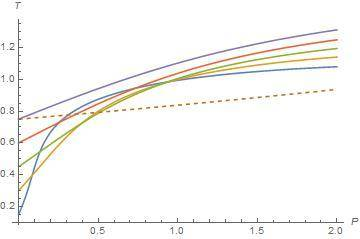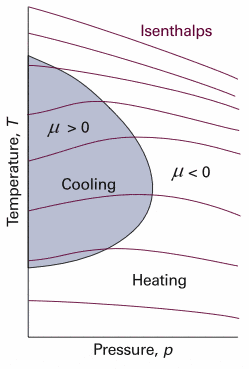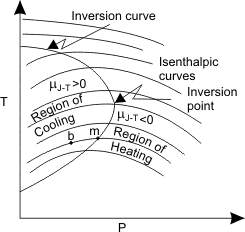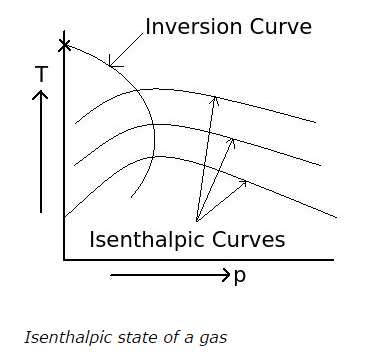# Inversion and Isenthelpic Curves for van der Waals EOS

• I
• ozgur(tr)

#### ozgur(tr)

When I plot the isenthalpic curves in T-P plane, I see isenthalpic curves are not well defined in the lower temperature region in T-P plane. Inside the inversion curve Joule-Thomson coefficient is positive so gas cools and outside of the inversion curve Joule-Thomson coefficent is negative so gas warms. Also we know heat capacity at constant pressure is always positive and change of pressure is always negative. It means that change of temperature plays a role on sign of Joule-Thomson coefficient. But below the lower inversion temperature, Joule-Thomson coefficient is positive in my plot. I see other T-P planes on the internet or in the textbooks but they are well behaved in the T-P plane. Joule-Thomson coefficient is negative below the lower curve. I am not sure whether other plotts are experimental or not. Maybe I made a mistake or van der Waals EOS is not well defined for lower inversion curve or low temperature isenthalpic curve. For example, isenthalpic curves are well defined in the top of the upper inversion curves in my plot. Here I share my plot and another plot.In my plot, dashed line corresponds to inversion curves and other lines correspond to isenthalpic curves. I only share a small region. Below the lower inversion curve (dashed line), slope are positive so Joule-Thomson cofficient is positive but it must negative. Is it my mistake or van der Waals EOS is not well defined in this region?

When I plot the isenthalpic curves in T-P plane, I see isenthalpic curves are not well defined in the lower temperature region in T-P plane. Inside the inversion curve Joule-Thomson coefficient is positive so gas cools and outside of the inversion curve Joule-Thomson coefficent is negative so gas warms. Also we know heat capacity at constant pressure is always positive and change of pressure is always negative. It means that change of temperature plays a role on sign of Joule-Thomson coefficient. But below the lower inversion temperature, Joule-Thomson coefficient is positive in my plot. I see other T-P planes on the internet or in the textbooks but they are well behaved in the T-P plane. Joule-Thomson coefficient is negative below the lower curve. I am not sure whether other plotts are experimental or not. Maybe I made a mistake or van der Waals EOS is not well defined for lower inversion curve or low temperature isenthalpic curve. For example, isenthalpic curves are well defined in the top of the upper inversion curves in my plot. Here I share my plot and another plot.View attachment 99580 View attachment 99581
In my plot, dashed line corresponds to inversion curves and other lines correspond to isenthalpic curves. I only share a small region. Below the lower inversion curve (dashed line), slope are positive so Joule-Thomson cofficient is positive but it must negative. Is it my mistake or van der Waals EOS is not well defined in this region?

Here I want to share an article and talk about my method. http://arxiv.org/abs/1402.1205 In the article, author gives a comprehensive study of van der Waals equation of state and usually he uses reduced thermodynamic variables. One can solve Eq.(56) to obtain V(h). There are two solution and they give the same result. If one substitutes one of these roots into Eq.(38) (Equation of state) and then solving Eq.(38) for t(p,h), one can obtain isenthalpic curves in T-P plane. One can plot inversion curves using Eq.(130a) and Eq.(130b). I use Mathematica program for my calculation and plotting. Here I share a pdf file to show my analysis.

#### Attachments

• method.pdf
178.5 KB · Views: 487
Here I want to share an article and talk about my method. http://arxiv.org/abs/1402.1205 In the article, author gives a comprehensive study of van der Waals equation of state and usually he uses reduced thermodynamic variables. One can solve Eq.(56) to obtain V(h). There are two solution and they give the same result. If one substitutes one of these roots into Eq.(38) (Equation of state) and then solving Eq.(38) for t(p,h), one can obtain isenthalpic curves in T-P plane. One can plot inversion curves using Eq.(130a) and Eq.(130b). I use Mathematica program for my calculation and plotting. Here I share a pdf file to show my analysis.
Yikes. That's a lot of arithmetic. I was hoping to see something fundamental, like, to begin with, the equation for the partial derivative of temperature with respect to pressure at constant enthalpy for a van der Waals gas.

I am really confused. I do not think so I miscalculate. Some author do not consider minimum inversion temperature. For example, https://goo.gl/Jt3dPv authors use saturation curve as inversion curve and called extended inversion curve. It is more reasonable than minimum inversion temperature. But in some textbooks and papers, authors give minimum inversion temperature and claim that real gas cools below the minimum inversion curves. One can obtain minimum and maximum inversion temperature by using van der Waals and other equation of states. But in my opinion minimum inversion temperature seems meaningless. I want to share two figures.In the first figure, minimum inversion temperature are considered but in the second, it is not considered and also both cases, authors usually avoid to plot the isenthalpic curves below the lower inversion curve.

I would like to take a step back, and get an idea of your level of understanding. In terms of temperature, pressure, and enthalpy, what is your understanding of the mathematical definition of the Joule Thompson coefficient? What is your understanding of the inversion curve? What is your understanding of the inversion point? What is the equation for dH, expressed as a function of dT and dP, for a van der Waals gas?

I don't know how you apporached this problem, but it seems different from the way that I would have approached it. The equation for dH is given by:
$$dH=C_pdT+\left[V-T\left(\frac{\partial V}{\partial T}\right)_P\right]dP$$
So, the Joule-Thompson coefficient is given by:
$$\mu=\left(\frac{\partial T}{\partial P}\right)_H=-\frac{\left[V-T\left(\frac{\partial V}{\partial T}\right)_P\right]}{C_p}$$
Along the inversion curve, the JT coefficient is equal to zero. So,$$T\left(\frac{\partial V}{\partial T}\right)_P=V$$
For a van der Waals gas, this reduces to:
$$P-\frac{a}{V^2}+\frac{2ab}{V^3}=\frac{RT}{V}\tag{1}$$
In addition to this equation, the van der Waals equation itself must be satisfied along the inversion curve:
$$\left(P+\frac{a}{V^2}\right)(V-b)=RT\tag{2}$$
Eqns. 1 and 2 can be regarded as two simultaneous linear algebraic equations for the two unknowns P and T, provided we regard P and T as being expressed parameterically in terms of the volume V. That is P = P(V) and T = T(V). So we can specify a value of V, and then solve Eqns. 1 and 2 for the corresponding P and T as the coordinates of a point along the inversion curve. By choosing a sequence of values for V, we can map out very simply then entire inversion curve.

All of this can be done much more conveniently by working in terms of reduced properties:
$$P_r=P/P_c$$
$$T_r=T/T_c$$
$$V^*=\frac{VP_c}{RT_c}$$
$$a^*=\frac{aP_c}{R^2T_c^2}\approxeq{\frac{27}{64}}$$
$$b^*=\frac{bP_c}{RT_c}\approxeq{\frac{1}{8}}$$

In terms of these dimensionless parameters, Eqns. 1 and 2 become:
$$P_r-\frac{a^*}{(V^*)^2}+\frac{2a^*b^*}{(V^*)^3}=\frac{T_r}{V^*}\tag{3}$$
$$\left(P_r+\frac{a^*}{(V^*)^2}\right)(V^*-b^*)=T_r\tag{4}$$
The advantage of this method is that you don't have to solve a non-linear equation for V to get the inversion curve.

The analytic solution I get is:
$$P_r=\frac{2a^*}{b^*V^*}\left(1-1.5\frac{b^*}{V^*}\right)$$
$$T_r=\frac{2a^*}{b^*}\left[\frac{V^*-b^*}{V^*}\right]^2$$

This solution is consistent with the intercept you obtained on your plot (reduced temperature = 0.75). It also predicts that, at the inversion point, the reduced temperature is about 3 and the reduced pressure is about 9. At very large volumes, the reduced pressure approaches 0, and the reduced temperature is about 6.75.

Last edited: